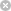RTXI  2.1rowvec< T > Class Template Reference

`#include <matrix_t.h>`

Inheritance diagram for rowvec< T >:## Public Member Functions

rowvec (void)

~rowvec (void)

rowvec (int origin, int size)

rowvec< T > & operator* (matrix< T > &m2)

rowvec< T > & operator= (vector< T > &vec)

colvec< T > & operator! ()

T & operator* (colvec< T > &v2)Public Member Functions inherited from vector< T >
vector (int origin, int size)

vector (vector< T > &x)

void PurgeData (void)

~vector (void)

vector< T > & operator= (vector< T > &vec)

vector< T > & operator= (T x)

vector< T > & operator/ (T x)

T * array (void)

T & operator[] (int)

vector< T > & operator* (matrix< T > &m2)

## Friends

class colvec< T >Protected Member Functions inherited from vector< T >
vector (void)Protected Attributes inherited from vector< T >
struct vector::vrepp

int Is_Temp

## Detailed Description

### template<class T>class rowvec< T >

Definition at line 6 of file matrix_t.h.

## Constructor & Destructor Documentation

template<class T >
 rowvec< T >::rowvec ( void )

Definition at line 20 of file vector_t.cpp.

template<class T >
 rowvec< T >::~rowvec ( void )

Definition at line 302 of file vector_t.cpp.

template<class T >
 rowvec< T >::rowvec ( int origin, int size )

Definition at line 31 of file vector_t.cpp.

## Member Function Documentation

template<class T >
 colvec< T > & rowvec< T >::operator! ( void )

Definition at line 67 of file vector_t.cpp.

template<class T>
 rowvec< T > & rowvec< T >::operator* ( matrix< T > & m2 )

Definition at line 133 of file vector_t.cpp.

template<class T>
 T & rowvec< T >::operator* ( colvec< T > & v2 )

Definition at line 90 of file vector_t.cpp.

template<class T>
 rowvec< T > & rowvec< T >::operator= ( vector< T > & vec )

Definition at line 446 of file vector_t.cpp.

## Friends And Related Function Documentation

template<class T>
 friend class colvec< T >
friend

Definition at line 73 of file vector_t.h.

The documentation for this class was generated from the following files: